NCERT Solutions for Class 7 Maths Chapter 9 Rational Numbers Ex 9.2 are part of NCERT Solutions for Class 7 Maths. Here we have given NCERT Solutions for Class 7 Maths Chapter 9 Rational Numbers Ex 9.2.

 Board CBSE Textbook NCERT Class Class 7 Subject Maths Chapter Chapter 9 Chapter Name Rational Numbers Exercise Ex 9.2 Number of Questions Solved 4 Category NCERT Solutions

## NCERT Solutions for Class 7 Maths Chapter 9 Rational Numbers Ex 9.2

Question 1.
Find the sum: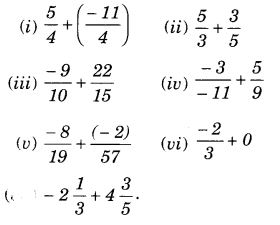Solution: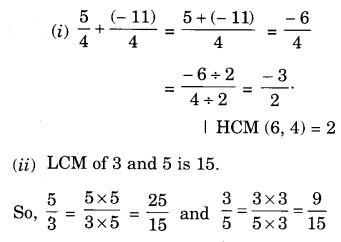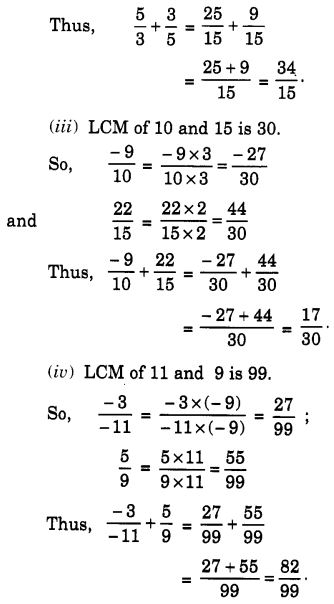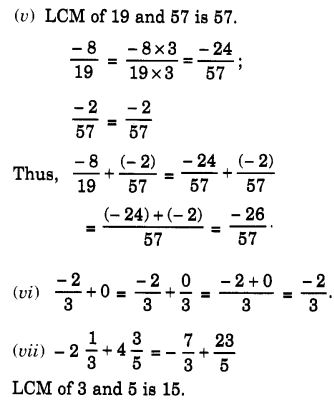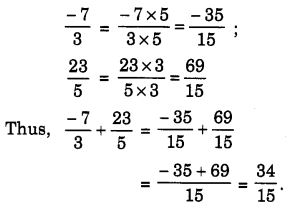Question 2.
Find :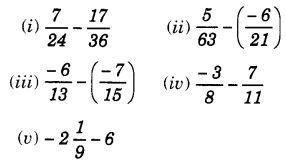Solution: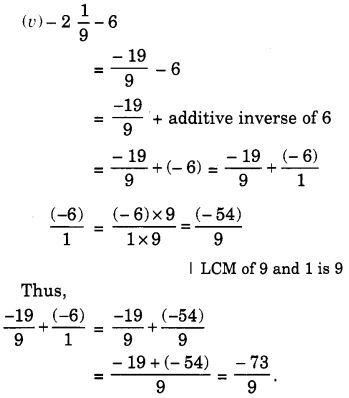Question 3.
Find the product :Solution: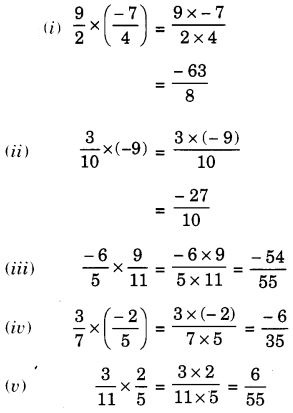Question 4.
Find the value of :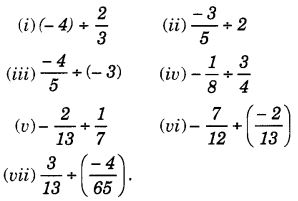Solution: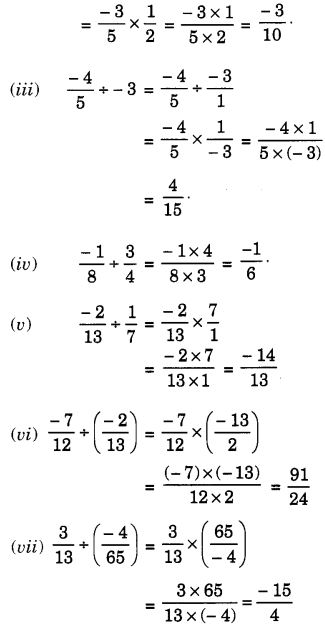We hope the NCERT Solutions for Class 7 Maths Chapter 9 Rational Numbers Ex 9.2 help you. If you have any query regarding NCERT Solutions for Class 7 Maths Chapter 9 Rational Numbers Ex 9.2, drop a comment below and we will get back to you at the earliest.